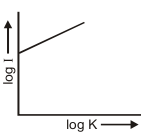Most Affordable JEE | NEET | 8,9,10 Preparation by Kota's Top IITian Doctor Faculties

# Radius of Gyration Class 11| Definition & Equation| JEE & NEET`
Radius of Gyration Class 11 As a measure of the way in which the mass of a rotating rigid body is distributed with respect to the axis of rotation, we define a new parameter known as the radius of gyration. It is the distance whose square when multiplied with the mass of the body gives the moment of inertia of the body about the given axis. For a body of mass $M,$ if $K$ is radius of gyration $\mathrm{I}=\mathrm{MK}^{2}$ $\mathrm{K}=\sqrt{\frac{\mathrm{I}}{\mathrm{M}}} \quad \mathrm{K}=\sqrt{\frac{\sum_{i=1}^{n} m_{i} r_{i}^{2}}{M}}$ Through this concept a real body (particular) is replaced by a point mass for dealing its rotational motion. e.g., in case of a solid sphere rotating about an axis through its centre of mass. $\mathrm{K}=\sqrt{\frac{\mathrm{I}}{\mathrm{M}}}=\sqrt{\frac{(2 / 5) \mathrm{MR}^{2}}{\mathrm{M}}}=\mathrm{R} \sqrt{\frac{2}{5}}$ So instead of solid sphere we can assume a point mass M at a distance $(\mathrm{R} \sqrt{\frac{2}{5}})$ from the axis of rotation for dealing the rotational motion of the solid sphere. K depends on (i) axis of rotation(ii) distribution of mass of body K does not depend on (i) Mass of the body $\quad$ (ii) Position of body (iii) On all angular physical quantity
• radius of gyration does not depends on the mass of rigid body. Graphical relation between log K and log I.Angular Momentum The moment of linear momentum of a moving particle with respect to axis of rotation is known as angular momentum. It is a vector quantity, which is often represented by $\overrightarrow{\mathrm{L}}$ or $\overrightarrow{\mathrm{J}}$. It is an axial vector (i.e. always perpendicular to the plane of motion) \begin{aligned} \text { Angular momentum } & \vec{J}=\overrightarrow{\mathrm{r}} \times \overrightarrow{\mathrm{p}} \\ &=\overrightarrow{\mathrm{r}} \times(\mathrm{m} \overrightarrow{\mathrm{v}})=\mathrm{m}(\overrightarrow{\mathrm{r}} \times \overrightarrow{\mathrm{v}}) \end{aligned} or $\quad \vec{J}=r p \sin \theta \hat{n}$ $\theta$ is angle between $\vec{r}$ and $\vec{v}$ $\hat{n}$ is unit vector perpendicular to plane of $\overrightarrow{\mathrm{r}}$ and $\overrightarrow{\mathrm{v}}$ The direction of angular momentum is perpendicular to the plane of $\overrightarrow{\mathrm{r}}$ and $\overrightarrow{\mathrm{v}}$ and it given by right hand screw rule. $\begin{array}{ll}{\text { If } \theta=0^{\circ},} & {J=0 \quad \text { (Minimum) }} \\ {\text { If } \theta=90^{\circ},} & {J=m \vee r \quad \text { (Maximum) }}\end{array}$ S.I. Unit of $J$ is Jule $\times$ sec (same as that of Planck's const.) Dimension: $\mathrm{M}^{1} \mathrm{L}^{2} \mathrm{T}^{-1}$ If direction of rotation is anticlockwise, angular momentum is taken positive and if direction of rotation is clockwise, angular momentum is taken negative. The angular momentum of a system of particles is equal to the vector sum of angular momentum of each particle $\vec{J}=\vec{J}_{1}+\vec{J}_{2}+\vec{J}_{3}+\ldots \ldots \ldots . \quad \vec{J}=\sum_{i=1}^{n} \vec{J}_{i}$
##### Relation between angular momentum and angular velocity
$J=I \omega$ I- Moment of inertia with respect to axis of rotation $\omega$ - Angular velocity due to angular momentum J- The moment of momentum which is causing rotational motion. The rate of change of angular momentum is equal to the torque applied on the body. $$\vec{\tau}=\frac{d \vec{J}}{d t}$$ In rotational motion angular momentum has equal importance as linear momentum in linear motion If torque acting of a particle is zero then $\vec{\tau}=0 \Rightarrow \frac{d \vec{J}}{d t}=0 \quad$ or $\quad \vec{J}=$ constant Which implies that the angular momentum remians conserved when no external torque acts on the body. LAW OF CONSERVATION OF ANGULAR MOMENTUM If no external torque is acting upon a body rotating about an axis, then the angular momentum of the body remains constant that is $\vec{J}=\overrightarrow{\mathrm{I} \omega}=\mathrm{constant}$ Proof: For a rigid body, rotating about a given axis. $\quad \vec{\tau}=1 \vec{\alpha}=\mathrm{I} \cdot \frac{\mathrm{d} \vec{\omega}}{\mathrm{dt}}$ Now, for a rigid body, rotating about a given axis, Iremains constant. $\vec{\tau}=\mathrm{I} \cdot \frac{\mathrm{d} \vec{\omega}}{\mathrm{dt}}=\frac{\mathrm{d}(\overrightarrow{\mathrm{I} \omega})}{\mathrm{dt}}=\frac{\mathrm{d} \overrightarrow{\mathrm{J}}}{\mathrm{dt}}$ If the external torque acting on the body, $\vec{\tau}=0$ $$\frac{d \overrightarrow{\mathrm{J}}}{d t}=0, \text { and hence } \overrightarrow{\mathrm{J}}=\text { constant. }$$ For the equation $\mathrm{J}=\mathrm{I} \omega,$ it can be seen that if $\mathrm{J}$ remains constant, $\omega$ increases when I decreases and vice versa. If I changed to $\mathrm{I}_{1}$, then, in the absence of an external torque, $\omega$ will change to $\omega_{1}$, such that $\mathrm{I}_{\mathrm{i}} \omega_{\mathrm{i}}=\mathrm{I}_{\mathrm{f}} \omega_{\mathrm{f}}$ This is the law of conservation of angular momentum.     Introduction to Rotational Dynamics  Moment of Inertia Moment of Inertia: Perpendicular and Parallel axis theorem Radius of Gyration Law of Conservation of Angular Momentum Conservation of Angular Momentum Examples Kinetic Energy of a Rotating Body Work done in rotatory Motion Rotational Power Combine Translational and Rotational Motion Rolling without slipping Rolling on a plane surface Rolling on a Inclined Plane   For Latest updates, Subscribe our Youtube Channel